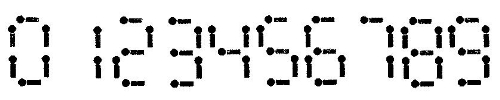### 最新评论：leijiankun 说：膜拜神牛。……css 说：康拓展开和逆康拓展……songrenchu 说：欢迎大家踊……Admin 说：……

# 【有道难题资格赛三】解题报告

## 发表时间：2010-05-31 13:29:52  浏览(5202) 评论(3)

A：选课的困惑

【题目叙述】

【输入】

1<=T<=100, 1 <= n,m <= 100, 0 <= ai, cj <= 100, 1 <= bi, dj <= 5，所有的分数小数点后至多两位。

【输出】

【样例输入】
2
3 4
80.00 1
40.00 2
20.00 2
90.00 3
88.00 2
92.00 2
100.00 1
3 4
62.00 1
70.00 1
80.00 1
99.00 2
100.00 2
100.00 2
100.00 2

【样例输出】
Impossible
3

【算法分析】

【我的代码】
var i,j,k,l,m,n,p,q,r,s,t,d:longint;
cg:array[0..1000] of double;
dt,mid,mm:double;
ans:longint;

begin
for d:=1 to t do
begin
dt:=0;
for i:=1 to n do
begin
dt:=dt+(mid-90)*k;
end;
for i:=1 to m do
begin
cg[i]:=(mid-90)*k;
end;
if (dt>=0) then
begin
writeln(0);
continue;
end;
for i:=1 to m-1 do
for j:=i+1 to m do
if (cg[i]<cg[j]) then
begin
mm:=cg[i];cg[i]:=cg[j];cg[j]:=mm;
end;
for i:=1 to m do
begin
dt:=dt+cg[i];
if (dt>=0) then break;
end;
if (dt>=0) then writeln(i) else writeln('Impossible');
end;
end.

【现场分析】

B：X星球的身份证系统

【题目叙述】

【输入】

【输出】

【样例输入】
3
bca

【样例输出】
28

【算法分析】

【我的代码】
var i,j,k,l,m,n,p,q,r,t,ans:longint;
c:array[0..30] of longint;
esl:array[0..30] of longint;
ch:char;
can:boolean;

function hw(x:longint):longint;
begin
exit(esl[x div 2+x mod 2]);
end;

begin
for i:=1 to n do
begin
c[i]:=ord(ch)-ord('a')+1;
end;
if (n=1) then
begin
writeln(c);
halt;
end;
esl:=1;
for i:=1 to 30 do esl[i]:=(esl[i-1]*26) mod 10000;
k:=n div 2;
ans:=0;can:=true;
for i:=1 to k do
begin
ans:=(ans+hw(n-i*2)*(c[i]-1)) mod 10000;
if (c[i]>c[n-i+1]) then can:=false;
if (c[i]<c[n-i+1]) then can:=true;
end;
if (n mod 2=0) then
begin
if (c[k]<=c[k+1]) then if can then ans:=(ans+1) mod 10000;
end else
begin
if (can) then
ans:=(ans+c[k+1]) mod 10000 else
ans:=(ans+c[k+1]-1) mod 10000;
end;
writeln(ans);
end.

【现场分析】

C：火柴游戏

【题目叙述】【输入】

T < =20,
N, M <=10000并且M*N<=1000000

【输出】

【样例输入】
4
4 15
20 4
6 10
6 20

【样例输出】
1
6982
1
2

【算法分析】
DP。dp[i,j]表示用了i个火柴，得到的数字模m等于j的情况总数。us[k]（k=0..9）表示摆k用的火柴数量。则我们把每个dp[i,j]加到dp[i+us[k],(j*10+k) mod m]上面。最终我们对dp[n,p]（p为小于m的质数）求和就是答案。复杂度O(T*N*M*10)

【我的代码】
const us:array[0..9] of longint=(6,2,5,5,4,5,6,3,7,6);
mo=1000000007;

var i,j,k,l,m,n,p,q,r,s,t,d,pp:longint;
dp:array[0..2000000] of longint;
prime:array[1..10000] of longint;
pre:array[0..10000,0..9] of longint;
oup:longint;

function pr(x:longint):boolean;
var g:longint;
begin
if (x<2) then exit(false);
if (x=2) then exit(true);
for g:=2 to trunc(sqrt(x)) do
if (x mod g=0) then exit(false);
exit(true);
end;

begin
pp:=0;
for i:=2 to 10000 do if (pr(i)) then
begin
inc(pp);prime[pp]:=i;
end;
for d:=1 to t do
begin
fillchar(dp,sizeof(dp),0);
for i:=1 to 9 do inc(dp[us[i]*m+i mod m]);
for i:=0 to m-1 do
for j:=0 to 9 do
pre[i,j]:=(i*10+j) mod m;
for i:=2 to n-2 do
for j:=0 to m-1 do
begin
p:=i*m+j;
if (dp[p]=0) then continue;
for k:=0 to 9 do
begin
q:=(i+us[k])*m+pre[j,k];
dp[q]:=(dp[q]+dp[p]) mod mo;
end;
end;

oup:=0;
for i:=1 to pp do
begin
if (prime[i]>=m) then break;
oup:=(oup+dp[n*m+prime[i]]) mod mo;
end;
writeln(oup);
end;
end.

【现场总结】

【整场比赛总揽】

# 网友评论：

1  Guest 于 2010-06-02 15:53:03 说：貌似那个C用Pascal写很有劣势。。相同的做法我用C++卡过去了的。。回复Guest：同感！膜拜Pascal过此题的牛人们！话说您是用两种语言做的比赛？比赛中同一个选手不同的题能使用不同的语言提交？膜拜神牛。 我用c++写了第一个。ok的。

# Guest 发表评论